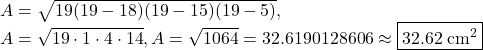## find the area of the triangle 15cm, 18cm, 5cm

Question

find the area of the triangle 15cm, 18cm, 5cm

in progress 0
2 weeks 2021-09-02T20:44:18+00:00 2 Answers 0 views 0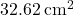Step-by-step explanation:

The area of a triangle with side lengths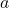,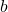, and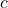is given by:

Let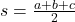(semi-perimeter)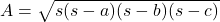We’re given three side lengths with lengths 15 cm, 18 cm, and 5 cm. Therefore, the semi-perimeter,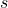, is equal to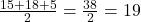.

Thus, the area of this triangle is: